# Samacheer Kalvi Books: Tamilnadu State Board Text Books Solutions

## Samacheer Kalvi 9th Maths Chapter 6 Trigonometry Notes PDF Download: Tamil Nadu STD 9th Maths Chapter 6 Trigonometry NotesSamacheer Kalvi 9th Maths Chapter 6 Trigonometry Notes PDF Download: Tamil Nadu STD 9th Maths Chapter 6 Trigonometry Notes

We bring to you specially curated Samacheer Kalvi 9th Maths Chapter 6 Trigonometry Notes PDF which have been prepared by our subject experts after carefully following the trend of the exam in the last few years. The notes will not only serve for revision purposes, but also will have several cuts and easy methods to go about a difficult problem.

 Board Tamilnadu Board Study Material Notes Class Samacheer Kalvi 9th Maths Subject 9th Maths Chapter Chapter 6 Trigonometry Format PDF Provider Samacheer Kalvi Books

## How to Download Samacheer Kalvi 9th Maths Chapter 6 Trigonometry Notes PDFs?

2. Click on the Samacheer Kalvi 9th Maths Notes PDF.
3. Look for your preferred subject.
4. Now download the Samacheer Kalvi 9th Maths Chapter 6 Trigonometry notes PDF.

## Tamilnadu Samacheer Kalvi 9th Maths Solutions Chapter 6 Trigonometry Additional Questions

Exercise 6.1

Question 1.
Find the six trigonometric ratios of the angle 6 using the diagram
Solution: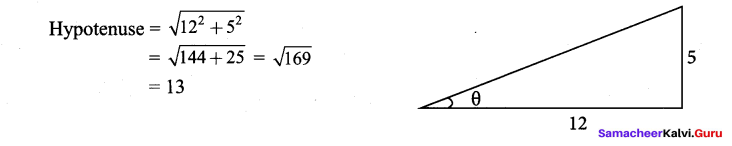Question 2.
If 3 cot θ = 1, then find the value of 3cosθ−4sinθ5sinθ+4cosθ
Solution: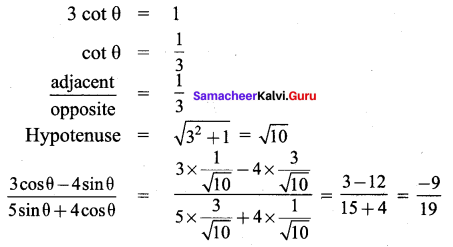Question 3.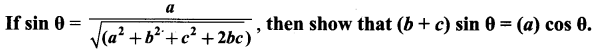Solution: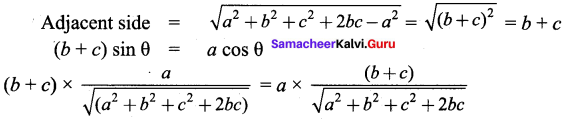Hence proved

Question 4.
If 3 (tan θ) + 4 (sec θ × sin θ) = 24. Then find all the trigonometric ratios of the angle θ.
Solution: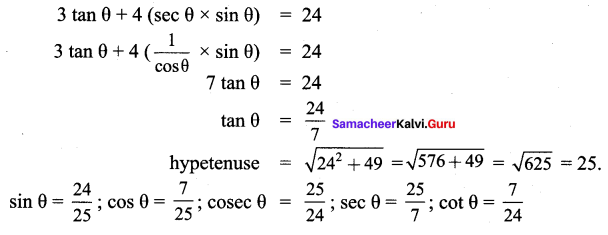Question 5.
From the given figure, find all the trigonometric ratios of angle θ.
Solution: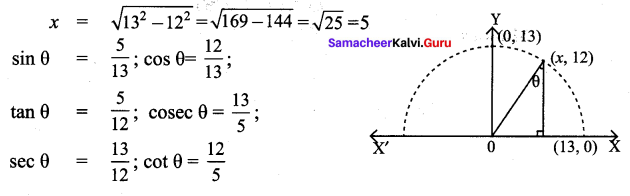Exercise 6.2

Question 1.
Find the value of sin 3x. sin 6x. sin 9x when x = 10°
Solution: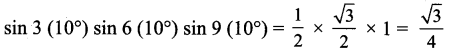Question 2.
Find the value of cot 15°. cot 30°. cot 45°. cot 60°. cot 75°
Solution:
cot (90° – 75) cot (90° – 60°) cot 45° cot 60° cot 75°
= tan 75° tan 60° (1) cot 60° cot 75° = 1

Exercise 6.3

Question 3.
Find the value of cos 19° 59′ + tan 12° 12′ + sin 49° 20′.
Solution:
cos 19° 59′ + tan 12° 12′ + sin 49° 20′ = 0

Question 4.
Given that sin α = 12√ and tan β = 3–√ . Find the value of α + β.
Solution: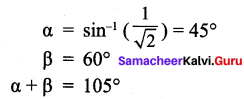Question 5.
Find the value of cos63∘20′sin26∘40′
Solution: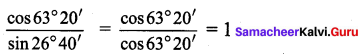## How to Prepare using Samacheer Kalvi 9th Maths Chapter 6 Trigonometry Notes PDF?

Students must prepare for the upcoming exams from Samacheer Kalvi 9th Maths Chapter 6 Trigonometry Notes PDF by following certain essential steps which are provided below.

• Use Samacheer Kalvi 9th Maths Chapter 6 Trigonometry notes by paying attention to facts and ideas.
• Pay attention to the important topics
• Refer TN Board books as well as the books recommended.
• Correctly follow the notes to reduce the number of questions being answered in the exam incorrectly
• Highlight and explain the concepts in details.

## Frequently Asked Questions on Samacheer Kalvi 9th Maths Chapter 6 Trigonometry Notes

#### How to use Samacheer Kalvi 9th Maths Chapter 6 Trigonometry Notes for preparation??

Read TN Board thoroughly, make separate notes for points you forget, formulae, reactions, diagrams. Highlight important points in the book itself and make use of the space provided in the margin to jot down other important points on the same topic from different sources.

#### How to make notes for Samacheer Kalvi 9th Maths Chapter 6 Trigonometry exam?

Read from hand-made notes prepared after understanding concepts, refrain from replicating from the textbook. Use highlighters for important points. Revise from these notes regularly and formulate your own tricks, shortcuts and mnemonics, mappings etc.
Share: